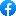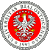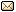XXXIX Workshop on Geometric Methods in Physics 19.06–25.06.2022 XI School on Geometry and Physics 27.06–1.07.2022
 Home First Announcement Second Announcement Travel and local information Participants of Workshop Participants of School Registration Photos Proceedings Previous Workshops EmailIn order to secure the right conditions for our Workshop we have decided to exceptionally move (only for this year's meeting) the site of our Workshop to the campus of our University in Białystok.

# Safiqul Islam

## A further analysis of a (2 + 1)-dimensional combined cosmological model in $f (R, T )$ gravity

This research is an extension of my published (2 + 1) dimensional cosmological models in $f(R, T )$ gravity with $\Lambda(R, T )$ (IOP Conf. Series: Journal of Physics: Conf. Series 1258 , (2019) 012026). A new class of cosmological model in $f (R, T )$ modified theories of gravity is studied, and hence we define the cosmological constant $\Lambda$ as a function of the trace of the stress-energy momentum tensor $T$ and the Ricci scalar $R$, and call such a model $\Lambda(R, T )$ gravity. Here we have specified a certain form of $\Lambda(R, T )$. $\Lambda(R, T )$ is also defined in the perfect fluid as well as dust case. We intend to search for a combined model that satisfies the equation of state for dark energy matter or quintessence matter or perfect matter fluid. Some geometric and physical properties of the model are also discussed. The pressure, density and energy conditions are studied both when $\Lambda$ is zero and when $\Lambda = \Lambda(r)$, a function of the radial parameter, $r$. We study the effective mass function and also the gravitational redshift function, both of which are found to be positive as per latest observations. The cosmological model is studied in $f (R, T )$ modified theory of gravity, where the gravitational Lagrangian is expressed both in terms of the Ricci scalar $R$ and the trace of the stress-energy tensor $T$. The equation of state parameter is discussed in terms of $\omega$ corresponding to the three cases mentioned above. The behaviour of the cosmological constant is separately examined in presence of quintessence matter, dark energy matter and perfect fluid matter.

 Event sponsored by: University of BialystokWebpage by: Tomasz Golinski